The PANEL Procedure

One-Way Fixed-Effects Model

The specification for the one-way fixed-effects model is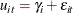where the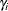s are nonrandom parameters to be estimated.

Let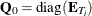, withand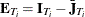, where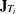is a matrix of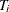ones.

The matrix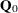represents the within transformation. In the one-way model, the within transformation is the conversion of the raw data to deviations from a cross section’s mean. The vector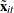is a row of the general matrix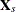, where the subscripted s implies the constant (column of ones) is missing.

Let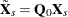and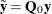. The estimator of the slope coefficients is given by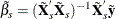Once the slope estimates are in hand, the estimation of an intercept or the cross-sectional fixed effects is handled as follows. First, you obtain the cross-sectional effects: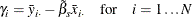If the NOINT option is specified, then the dummy variables’ coefficients are set equal to the fixed effects. If an intercept is desired, then the ith dummy variable is obtained from the following expression: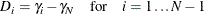The intercept is the Nth fixed effect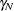.

The within model sum of squared errors is: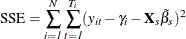The estimated error variance can be written: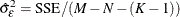Alternatively, an equivalent way to express the error variance is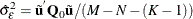where the residuals
are given by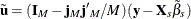if there is an intercept and by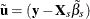if there is not. The drawback is that the formula changes (but the results do not) with the inclusion of a constant.

The variance covariance matrix of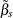is given by: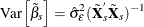The covariance of the dummy variables and the dummy variables with theis dependent on whether the intercept is included in the model.

• no intercept: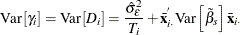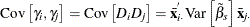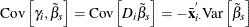• intercept: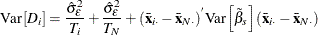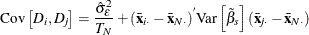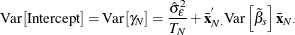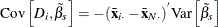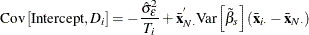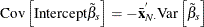Alternatively, the model option FIXONETIME estimates a one-way model where the heterogeneity comes from time effects. This option is analogous to re-sorting the data by time and then by cross section and running a FIXONE model. The advantage of using the FIXONETIME option is that sorting is avoided and the model remains labeled correctly.